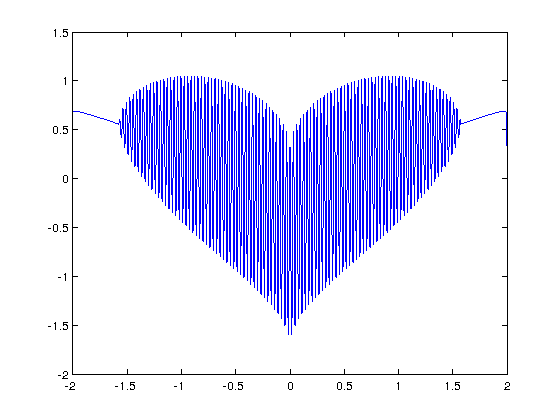## How to plot a heart

December 31st, 2011 | Categories: just for fun, mathematica, matlab | Tags:

I’ve seen several equations that plot a heart shape over the years but a recent google+ post by Lionel Favre introduced me to a new one.  I liked it so much that I didn’t want to wait until Valentine’s day to share it.  In Mathematica:

Plot[Sqrt[Cos[x]]*Cos[200*x] + Sqrt[Abs[x]] - 0.7*(4 - x*x)^0.01, {x, -2, 2}]

and in MATLAB:

>> x=[-2:.001:2];
>> y=(sqrt(cos(x)).*cos(200*x)+sqrt(abs(x))-0.7).*(4-x.*x).^0.01;
>> plot(x,y)
Warning: Imaginary parts of complex X and/or Y arguments ignored

The result from the MATLAB version is shown belowUpdate

Rene Grothmann has looked at this equation in a little more depth and plotted it using Euler.

Similar posts

1. Actually this is a way better link. It has equations for all sorts of pastas
http://www.nytimes.com/interactive/2012/01/10/science/20120110_pasta.html

2. The MATLAB version above is missing assignment of the “x” variable. This command for “x” seems to work well:

>> x=[-2:.001:2];

3. Thanks Scott, I’ve updated the main text.

4. Sorry for being late to reply. I tried it in MathStudio (previously known as TimeSpace Mathematics) for Android. FINALLY we have a very good math application for android. It’s too pricey though since it’s $20 dollars compared to$10 dollars for the iPhone. I hope the price goes down though.

Here’s the screenshots of the heart!
http://imgur.com/a/c7wja

5. Thanks for that Silver. MathStudio is a great piece of software–I’ve bought it several times now! First for Ye Olde Windows Mobile, then for iPad and finally for Android.

6. Very cool! I took the liberty of animating it a bit :)

http://i.imgur.com/NjM21.gif

Export[Environment[“userprofile”] “\\heart.gif”,
Table[
Plot[Sqrt[Cos[d*x]]*Cos[153*d*x] + Sqrt[Abs[d*x]] – 0.7*(4 – x*x)^0.01, {x, -2, 2}, PlotStyle -> Red],
{d, 1.3, 1.8, 0.1}
] // {#, Reverse[#]} & // Flatten
]

7. Here is the python version for those interested

import numpy as np
import matplotlib.pyplot as plt

x=np.r_[-2:2:0.001]
y=(np.sqrt(np.cos(x))*np.cos(200*x)+np.sqrt(np.abs(x))-0.7)*np.power((4-x*x),0.01)
plt.plot(y)
plt.savefig(‘Heart’)

8. I am wondering how to plot a 3D heart by mesh function in MATLAB…

9. Hi Qing

Try the code at

http://www.walkingrandomly.com/?p=455

There are two pieces of code, A star and a heart.

10. plot(x,real(y)) not warning ;) regards

11. ending with plot(x,y,’r’) could be more better